# The Factorial function

We explain Factorial growth step by step. Learn how to use and calculate the Factorial formula easily.

## What is the Factorial function?

The factorial function is a mathematical formula represented by an exclamation mark "!". In the Factorial formula, you must multiply all the integers and positives that exist between the number that appears in the formula and the number 1.

Here’s an example:

``7! = 1 * 2 * 3 * 4 * 5 * 6 * 7 = 5.040``

On this formula, number 7 will be called the 7th factorial and multiplied by all the numbers that appear on the example until number 1.

If at this stage you are about to give up I will confess that in your calculator appears a button that will calculate the factorial of the number that you want automatically. You should look for a button similar to "x!" Or "n!".

## Examples of factorial formulas

``````1! = 1 * 1 = 1
3! = 1 * 2 * 3 = 6
10! = 1 * 2 * 3 … 8 * 9 * 10 = 3.628.800``````

What about the 0 factorial, how to calculate it? If we go back to the definition of factorial function we can see that it does not make sense to apply it in the case of "0". There are no positive numbers before 0 so 0 x 0 = 0.

However, it has been agreed that in the case of 0 factorial the result will be equal to 1:

``0! = 0 x 0 = 1``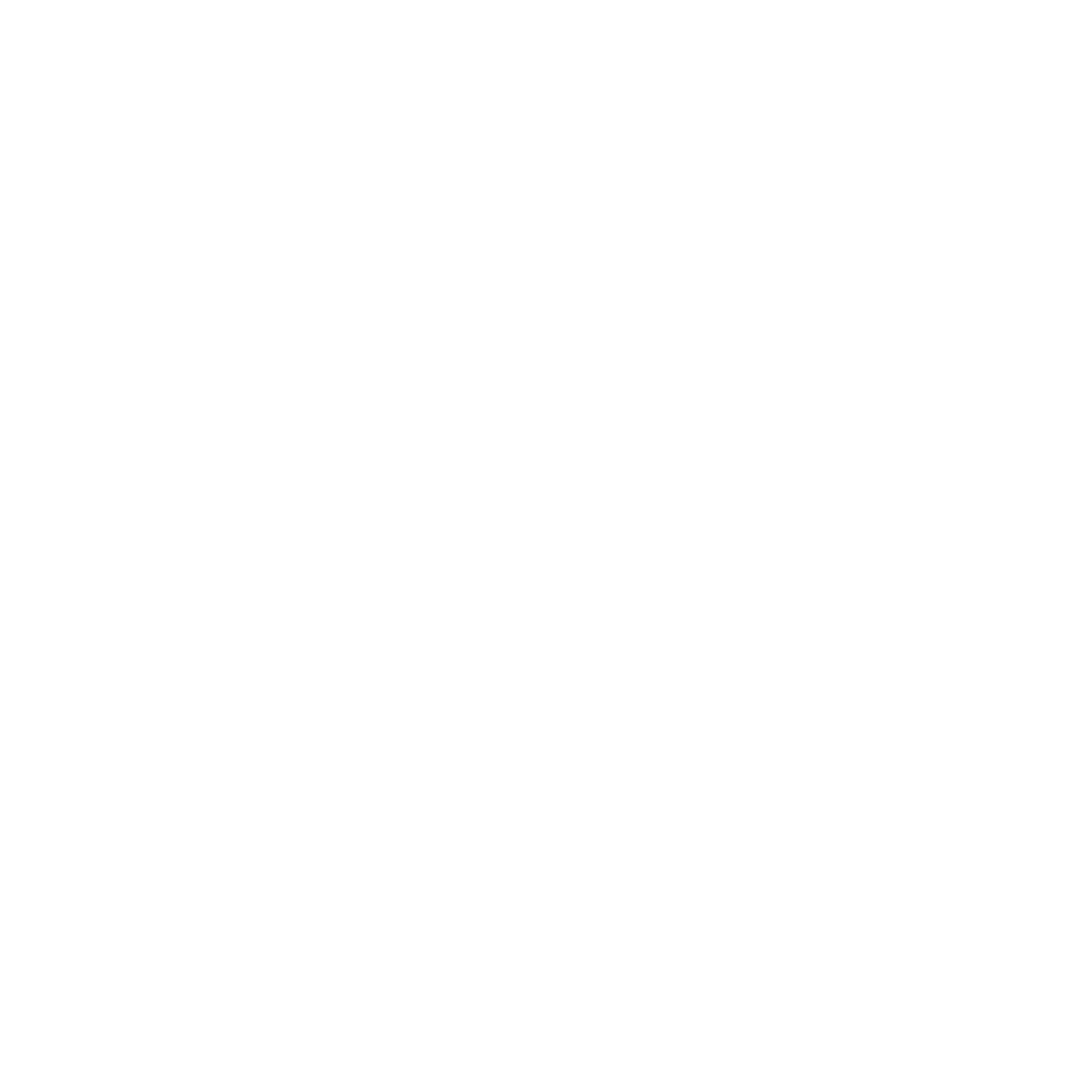## The utility of the Factorial function

It is common to use Factorial functions to calculate combinations and permutations. Thanks to the Factorial you can also calculate probabilities.

For example:

If we have 4 colored pictures and want to hang them on the wall, one after another we can calculate the number of possible combinations:

``4! = 1 * 2 * 3 * 4 = 24 possible combinations``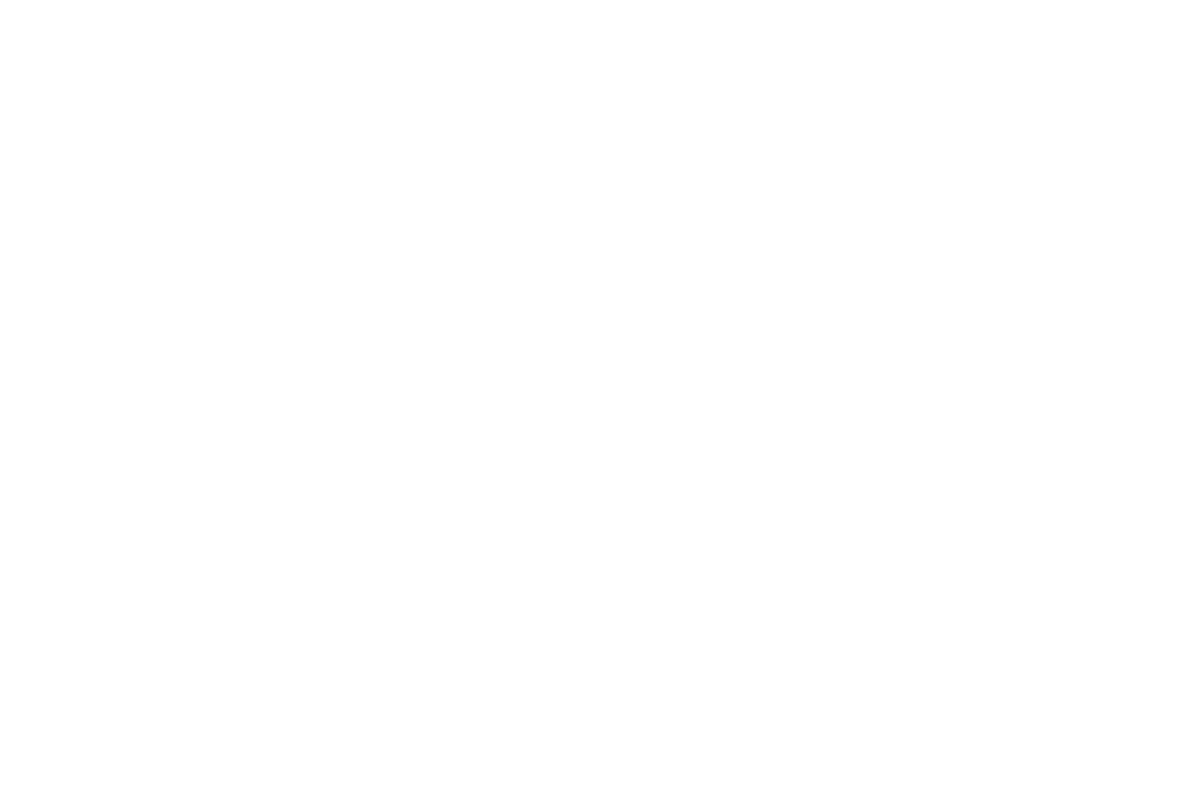## Why are we called Factorial?

At this point, you may be wondering why Factorial chose that name for its human resources software. Well, Factorial represents growth to the last power, a factorial growth of time, resources, productivity ... Factorial's human resources software allows the company to optimize its resources to keep growing factorially!

## Try it for free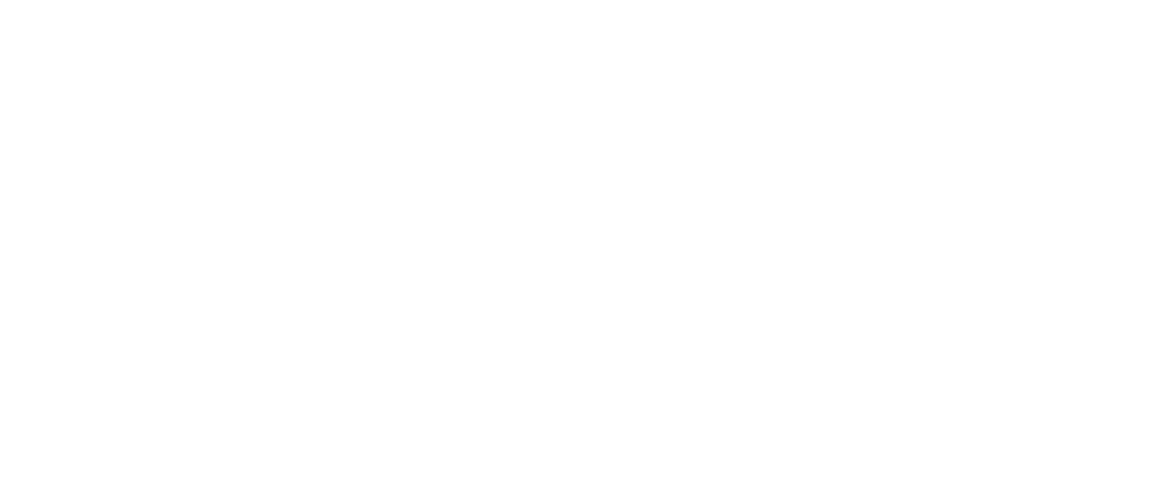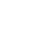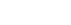United States